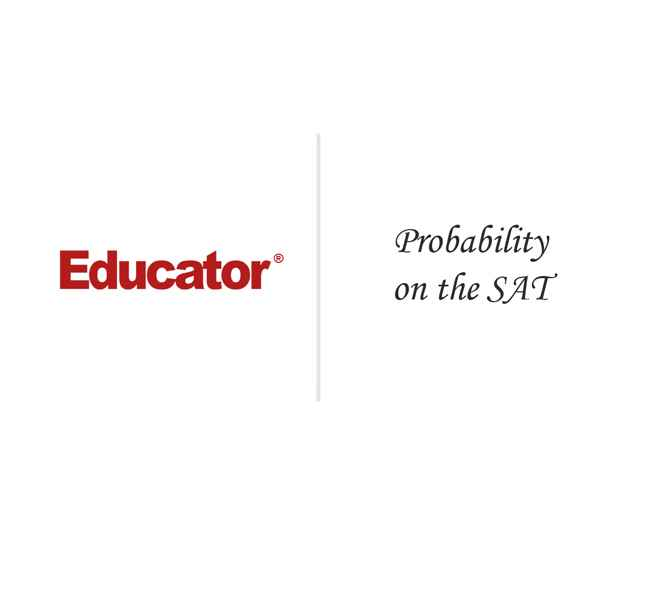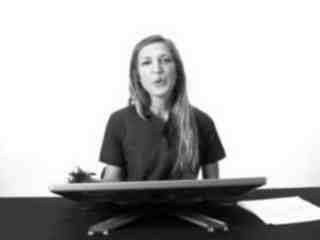Charlotte Vilkus

Probability on the SAT

Slide Duration:

Section 1: Basic Math
SAT Properties of Integers

6m 40s

Intro
0:00
How Properties of Integers are Tested
0:12
Learn by Example: Prime Factors
4:38
Fractions on the SAT

8m 50s

Intro
0:00
How Fractions are Tested on the SAT
0:11
Learn by Example: Evaluation with Fractions
3:16
Learn by Example: Word Problem with Fractions
6:32
Percent on the SAT

22m 16s

Intro
0:00
How the SAT Tests Percent
0:10
Percent Increase and Decrease
3:24
Percent More Than, Percent Less Than
7:53
Learn by Example: Percent Translation
9:29
Learn by Example: Percent More Than
13:38
Learn by Example: Dealing with Tax and Tip
16:31
Number Lines on the SAT

8m 31s

Intro
0:00
How Number Lines are Tested on the SAT
0:08
How to Solve SAT Number Line Problems
0:54
Learn by Example: Number Line Coordinates
4:52
Learn by Example: Representing and Inequality with a Number Line
6:33
Exponents on the SAT

9m 32s

Intro
0:00
How Exponents are Tested on the SAT
0:09
Consider Plugging in on Exponent Problems
0:21
Learn by Example: Exponents in Simultaneous Equations
1:03
Learn by Example: Exponent Analysis
6:15
Absolute Value on the SAT

12m 36s

Intro
0:00
How Absolute Value is Tested on the SAT
0:11
Learn by Example: Absolute Value
3:07
Learn by Example: Absolute Value and Inequalities
7:09
Section 2: Proportions, Rates and Variations
Proportions on the SAT

11m 15s

Intro
0:00
How Proportions are Tested on the SAT
0:11
Learn by Example: Map Scaling
2:07
Learn by Example: Medium to Hard Unit Conversion
4:40
Learn by Example: Where the SAT Really Tries to Trip You Up on Units
8:24
Rates on the SAT

12m 39s

Intro
0:00
How Rates are Tested on the SAT
0:12
Learn by Example: Videos Processed per Hour
2:50
Learn by Example: Multiple Rates
7:27
Variations on the SAT

7m 59s

Intro
0:00
How Variations are Tested on the SAT
0:13
Learn by Example: Direct Variation
2:22
Learn by Example: Variations
4:12
Learn by Example: Inverse Variation
7:04
Section 3: Geometry
Area and Perimeter on the SAT

16m 59s

Intro
0:00
SAT Problems on Area and Perimeter
0:11
Learn by Example: Perimeter of a Quarter Circle
2:50
Learn by Example: Area of the Unshaded Region
4:26
Learn by Example: Perimeter of a Square Inscribed in a Circle
10:03
Learn by Example: Using Pythagorean to Get Side Lengths
13:23
Angles on the SAT

12m 11s

Intro
0:00
How Angles are Tested on the SAT
0:11
What You Need to Know About Angles
1:13
Lean by Example: Angles (1)
2:48
Lean by Example: Angles (2)
5:45
Triangles on the SAT

15m 25s

Intro
0:00
What Sort of Triangles Will I see on the SAT?
0:11
Learn by Example: Triangle Basics
5:09
Learn by Example: Special Triangles
10:14
Learn by Example: Triangles with other Shapes
12:12

13m 34s

Intro
0:00
How Quadrilaterals are Tested on the SAT
0:12
Learn by Example: Squares
1:50
Learn by Example: Rectangle with Triangles
6:29
Learn by Example: Angles in a Quadrilateral Problem
11:17
Circles on the SAT

16m 40s

Intro
0:00
How Circles are Tested on the SAT
0:11
But Don't Bother with Any of This
1:40
How to Deal with Sections of a Circle
1:55
College Board Expects Mistakes Here
4:04
Circumference=Perimeter
4:47
Learn by Example: How Circle Problems Are Presented
5:18
Three Tangential Circles
5:48
Learn by Example: Concentric Circles
8:56
Learn by Example: Shaded Area with Combination Circles and Squares
11:53
Section 4: Graphing
SAT Coordinate Geometry

14m 38s

Intro
0:00
How the SAT Test Coordinate Geometry
0:17
Learn by Example: Midpoint and Distance
3:08
Distance Example: the Length of the Square
5:39
Example: Figures in the Coordinate Plane
9:34
SAT Translation

10m 6s

Intro
0:00
How the SAT Tests Graph Translation
0:11
Shifting the Graph in the Y: f(x)+a
1:01
Shifting the Graph in the X: f(x+a)
1:50
Multiplying the Graph af(x)
2:59
Multiplying the Graph f(ax)
5:07
How the SAT Tests Rotations and Reflections
6:19
Learn by Example: Rotations and Reflections
7:52
Lines on the SAT

6m 15s

Intro
0:00
How Lines are Tested on the SAT
0:09
Learn by Example: Finding the Intercept
1:48
Learn by Example: Perpendicular Lines
3:42
Line Slope on the SAT

10m 46s

Intro
0:00
How Line Slope is Tested on the SAT
0:10
Definition of Slope
0:25
Slope Equation
1:12
Classifying Slopes: Steep, Flat, Negative
1:50
Determining the Slope in Problems
3:41
Learn by Example: Line Slope (I)
4:43
Learn by Example: Line Slope (2)
8:04

6m 46s

Intro
0:00
How the SAT Tests Quadratic Equations
0:11
2:52
5:02
Expressions on the SAT

10m 20s

Intro
0:00
How Expression are Tested on the SAT
0:10
Learn by Example: Expression Evaluation--Signed Numbers and Exponents
1:17
Learn by Example: Expression Evaluation of Arithmetic Overload
6:16
Systems of Equations

28m 57s

Intro
0:00
The Basics: Systems of Equations
0:19
Equations with Infinite Solutions
0:44
Number of Equations Should Match the Number of Unknowns
2:41
Manipulation and Combination
4:34
Manipulation and Combination: Really?
7:23
Manipulation and Combination: Coefficients
10:41
Manipulation and Combination: Steps
13:50
Substitution
15:49
Which Method Should Be Used?
20:13
Oh No, Three Equations?
22:58
Three Equation Example
24:22
Inequalities on the SAT

13m 8s

Intro
0:00
How Inequalities are Tested on the SAT
0:11
What to Know: Inequalities
3:12
Learn by Example: Solving an Inequality for a Variable
7:12
Learn by Example: Inequalities with Parameters
9:02
Special Functions on the SAT

8m 16s

Intro
0:00
'Special Functions' on the SAT
0:09
Treating Special Functions like General Function Evaluation *Same Idea (Implies)
1:24
Learn by Example: Special Functions (1)
3:40
Learn by Example Special Functions: (2)
4:40
Section 5: Statistics, Combinations and Probability
Averages on the SAT

10m 20s

Intro
0:00
How Averages are Tested on the SAT
0:10
Important Quantities: Average, Sum and Number of Values
0:36
Rearranging the Average Equation to be of Maximum Use
1:36
Learn by Example: Averages (1)
3:16
Learn by Example: Averages (2)
7:17
Median and Mode on the SAT

8m 31s

Intro
0:00
How the SAT Test Median and Mode
0:32
Learn by Example: Using a Table to find the Median
1:46
Learn by Example: Finding the Median with an Unknown
4:41
Learn by Example: Mode
6:35
Data Analysis on the SAT

18m

Intro
0:00
How Data Analysis is Tested on the SAT
0:11
Tables
1:16
Using Table Totals
2:08
Pie Charts
3:50
Pie Charts Examples
5:56
Creating Pie Charts
7:00
Bar Graphs
9:35
Comparing Pie Charts and Bar Graphs
11:22
Scatter Plot Graphs
12:45
Example of a Scatter Plot
13:48
Line Graphs
15:12
Line Chart Example
15:32
Probability on the SAT

9m 37s

Introduction
0:00
How the SAT Tests Probability
0:10
Probability Definition
0:54
Probability of A or B occurring
1:56
Probability of A and B both occurring
3:16
Learn by Example: Probability (1)
4:12
Learn by Example: Probability (2)
6:07
Combinations and Permutations on the SAT

10m 10s

Intro
0:00
How Combinations and Permutations are Tested on the SAT
0:14
Using 'Blank Space' Representors
1:32
Learn by Example: Combinations
2:44
Writing Out the Possibilities
3:53
Learn by Example: Permutations
5:14
Writing Out the Possibilities
7:04
Logical Reasoning on the SAT

9m 18s

Intro
0:00
How Logical Reasoning is Tested on the SAT
0:11
Learn by Example: Logic Questions and Using Differences
0:54
Learn by Example: Logic Questions with Inequalities
5:32
Bookmark & Share Embed

## Copy & Paste this embed code into your website’s HTML

Please ensure that your website editor is in text mode when you paste the code.
(In Wordpress, the mode button is on the top right corner.)
×
• - Allow users to view the embedded video in full-size.
Since this lesson is not free, only the preview will appear on your website.

• ## Related Books & Services0 answersPost by Jeffrey Tao on October 26, 2013This is more of an important point for other viewers than a question. In the case of the crayons, the probability of picking in the AND case is 1/10 x 1/10 ONLY if after one crayon is picked, it is put back into the box before the second pick. Otherwise, if the crayon is left out, the probability is 1/10 x 1/NINE because if the crayon isn't put back after the first draw, then during the second time picking the crayon, there will be only nine crayons, thus it is 1/NINE x 1/10.

### Probability on the SAT

Lecture Slides are screen-captured images of important points in the lecture. Students can download and print out these lecture slide images to do practice problems as well as take notes while watching the lecture.

• Introduction 0:00
• How the SAT Tests Probability 0:10
• Probability Definition
• Probability of A or B occurring
• Probability of A and B both occurring
• Learn by Example: Probability (1) 4:12
• Learn by Example: Probability (2) 6:07

OR

### Start Learning Now

Our free lessons will get you started (Adobe Flash® required).